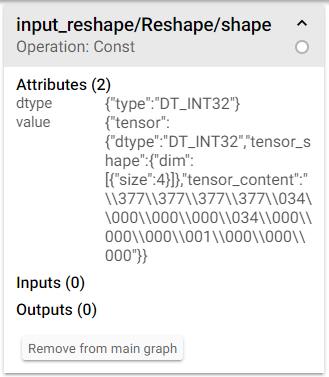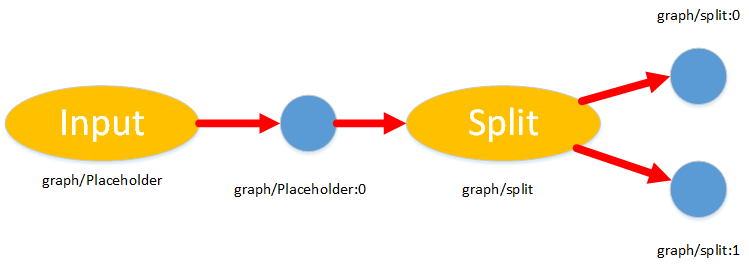Jarvis' Blog (总有美丽的风景让人流连) 总有美丽的风景让人流连

# Tensorflow 模型的保存、读取和冻结、执行

2018-04-25
2018-12-26
Jarvis
Post

checkpoint
model.ckpt.data-00000-of-00001
model.ckpt.index
model.ckpt.meta

Tensorflow 模型冻结是指把计算图的定义模型权重合并到同一个文件中, 可以按照以下步骤实施:

• 恢复已保存的计算图: 把预先保存的计算图(meta graph) 载入到默认的计算图中, 并将计算图序列化.
• 加载权重: 开启一个会话(Session), 把权重载入到计算图中
• 删除推导所需以外的计算图元数据(metadata): 冻结模型之后是不需要训练的, 所以只保留推导(inference) 部分的计算图 (这部分可以通过指定模型输出来自动完成)
• 保存到硬盘: 序列化冻结的 graph_def 协议缓冲区(Protobuf) 并转储到硬盘

## 1. 模型的保存

TF 使用 `saver = tf.train.Saver()` 定义一个存储器对象, 然后使用 `saver.save()` 函数保存模型. `saver` 定义时可以指定需要保存的变量列表, 最大的检查点数量, 是否保存计算图等. 官网例子如下:

``````1
2
3
4
5
6
7
8
9
10
11
12
v1 = tf.Variable(..., name='v1')
v2 = tf.Variable(..., name='v2')

# 使用字典指定要保存的变量, 此时可以为每个变量重命名(保存的名字)
saver = tf.train.Saver({'v1': v1, 'v2': v2})

# 使用列表指定要保存的变量, 变量名字不变. 以下两种保存方式等价
saver = tf.train.Saver([v1, v2])
saver = tf.train.Saver({v.op.name: v for v in [v1, v2]})

# 保存相应变量到指定文件, 如果指定 global_step, 则实际保存的名称变为 model.ckpt-xxxx
saver.save(sess, "./model.ckpt", global_step)
``````

## 2. 模型的读取

TF 模型的读取分为两种, 一种是我们仅读取模型变量, 即 `index` 文件和 `data` 文件; 另一种是读取计算图. 通常来说如果是我们自己保存的模型, 那么完全可以设置 `saver.save()` 函数的 `write_meta_graph` 参数为 `False` 以节省空间和保存的时间, 因为我们可以使用已有的代码直接重新构建计算图. 当然如果为了模型迁移到其他地方, 则最好同时保存变量和计算图.

### 2.1 读取计算图

#### 2.1.1 读取计算图核心函数

`meta` 文件读取计算图使用 `tf.train.import_meta_graph()` 函数, 比如:

``````1
2
with tf.Session() as sess:
new_saver = tf.train.import_meta_graph("model.ckpt.meta")
``````

``````1
2
with tf.Session() as sess:
new_saver = tf.train.import_meta_graph("model.ckpt.meta", clear_devices=True)
``````

2.1 节剩下的内容我们尝试探索一下 TF 中图的一些内容和基本结构, 不感兴趣可以跳过直接看 2.2 节.

#### 2.1.2 获取计算图内的任意变量/操作

``````1
2
3
4
5
6
7
8
sess.graph.get_all_collection_keys()
# 或
sess.graph.collections
# 或
tf.get_default_graph().get_all_collection_keys()

# 输出
['summaries', 'train_op', 'trainable_variables', 'variables']
``````

``````1
2
3
4
from pprint import pprint
pprint(sess.graph.get_collection("summaries"))
pprint(sess.graph.get_collection("variables"))
...
``````

``````1
2
3
4
sess.graph.get_operations()
# 或
for op in sess.graph.get_operations():
print(op.name, op.values())
````````````1
sess.graph.get_operation_by_name("input_reshape/Reshape/shape").node_def
``````

### 2.2 读取模型变量

#### 2.2.1 读取模型变量核心函数

``````1
2
3
4
5
6
# 首先定义一系列变量
...
# 载入变量的值
saver = tf.train.Saver()
with tf.Session() as sess:
saver.restore(sess, "path/to/model.ckpt")
``````

#### 2.2.2 获取任意模型变量的属性

``````1
2
3
ckpt = tf.train.get_checkpoint_state(log_dir)
if ckpt and ckpt.model_checkpoint_path:
saver.restore(sess, ckpt.model_checkpoint_path)
``````

``````1
2
3
4
5
6
7
8
9
10
11
12
13
14
from tensorflow.python import pywrap_tensorflow as pt
# 获取 变量名: 形状
for k in sorted(vars):
print(k, vars[k])

# 获取 变量名: 类型
for k in sorted(vars):
print(k, vars[k])

# 获取张量的值
``````

``````1
2
3
4
5
6
7
8
9
10
11
12
13
14
15
from tensorflow.python.tools import inspect_checkpoint as chkp

# 打印检查点所有的变量
chkp.print_tensors_in_checkpoint_file("/tmp/model.ckpt", tensor_name='', all_tensors=True)

# tensor_name:  v1
# [ 1.  1.  1.]
# tensor_name:  v2
# [-1. -1. -1. -1. -1.]

# 仅打印检查点中的 v1 变量
chkp.print_tensors_in_checkpoint_file("/tmp/model.ckpt", tensor_name='v1', all_tensors=False)

# tensor_name:  v1
# [ 1.  1.  1.]
``````

## 3. 模型的冻结

model.ckpt.data-00000-of-00001
model.ckpt.index
model.ckpt.meta

``````1
2
3
4
5
6
7
8
9
10
11
12
13
14
15
16
17
18
19
20
import tensorflow as tf

# 指定模型输出, 这样可以允许自动裁剪无关节点. 这里认为使用逗号分割
output_nodes = ["Accuracy/prediction", "Metric/Dice"]

# 1. 加载模型
saver = tf.train.import_meta_graph("model.ckpt.meta", clear_devices=True)

with tf.Session(graph=tf.get_default_graph()) as sess:
# 序列化模型
input_graph_def = sess.graph.as_graph_def()
# 2. 载入权重
saver.restore(sess, "model.ckpt")
# 3. 转换变量为常量
output_graph_def = tf.graph_util.convert_variables_to_constants(sess,
input_graph_def,
output_nodes)
# 4. 写入文件
with open("frozen_model.pb", "wb") as f:
f.write(output_graph_def.SerializeToString())
``````

## 4. 模型的执行

``````1
2
3
4
5
6
7
8
# 读取模型并保存到序列化模型对象中
with open(frozen_graph_path, "rb") as f:
graph_def = tf.GraphDef()
# 导入计算图
graph = tf.Graph()
with graph.as_default():
tf.import_graph_def(graph_def, name="MyGraph")
``````

``````1
2
3
4
x_tensor = graph.get_tensor_by_name("MyGraph/input/image-input:0")
y_tensor = graph.get_tensor_by_name("MyGraph/input/label-input:0")
keep_prob = graph.get_tensor_by_name("MyGraph/dropout/Placeholder:0")
y_target_tensor = graph.get_tensor_by_name("MyGraph/accuracy/accuracy:0")
````````````1
2
3
4
5
6
7
8
9
10
11
12
from tensorflow.examples.tutorials.mnist import input_data